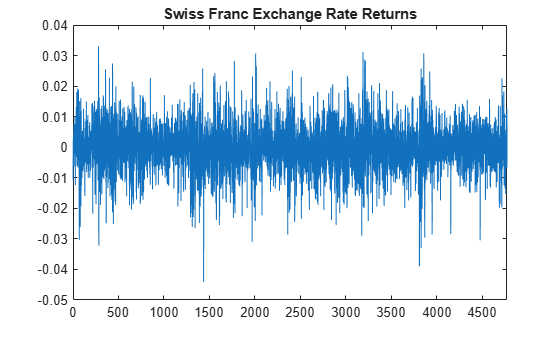Documentation

This is machine translation

Mouseover text to see original. Click the button below to return to the English version of the page.

Compare Conditional Variance Models Using Information Criteria

This example shows how to specify and fit a GARCH, EGARCH, and GJR model to foreign exchange rate returns. Compare the fits using AIC and BIC.

Load the foreign exchange rate data included with the toolbox. Convert the Swiss franc exchange rate to returns.

```load Data_FXRates y = DataTable.CHF; r = price2ret(y); T = length(r); logL = zeros(1,3); % Preallocate numParams = logL; % Preallocate figure plot(r) xlim([0,T]) title('Swiss Franc Exchange Rate Returns')```The returns series appears to exhibit some volatility clustering.

Step 2. Fit a GARCH(1,1) model.

Specify, and then fit a GARCH(1,1) model to the returns series. Return the value of the loglikelihood objective function.

```Mdl1 = garch(1,1); [EstMdl1,EstParamCov1,logL(1)] = estimate(Mdl1,r);```
``` GARCH(1,1) Conditional Variance Model (Gaussian Distribution): Value StandardError TStatistic PValue __________ _____________ __________ __________ Constant 1.6306e-06 4.3772e-07 3.7251 0.00019522 GARCH{1} 0.91369 0.0068888 132.63 0 ARCH{1} 0.058536 0.0050022 11.702 1.244e-31 ```
`numParams(1) = sum(any(EstParamCov1)); % Number of fitted parameters`

Step 3. Fit an EGARCH(1,1) model.

Specify, and then fit an EGARCH(1,1) model to the returns series. Return the value of the loglikelihood objective function.

```Mdl2 = egarch(1,1); [EstMdl2,EstParamCov2,logL(2)] = estimate(Mdl2,r);```
``` EGARCH(1,1) Conditional Variance Model (Gaussian Distribution): Value StandardError TStatistic PValue _________ _____________ __________ __________ Constant -0.29251 0.045942 -6.3669 1.9289e-10 GARCH{1} 0.96976 0.0046786 207.28 0 ARCH{1} 0.12292 0.012052 10.199 2.0094e-24 Leverage{1} -0.013229 0.0049498 -2.6727 0.007525 ```
`numParams(2) = sum(any(EstParamCov2));`

Step 4. Fit a GJR(1,1) model.

Specify, and then fit a GJR(1,1) model to the returns series. Return the value of the loglikelihood objective function.

```Mdl3 = gjr(1,1); [EstMdl3,EstParamCov3,logL(3)] = estimate(Mdl3,r);```
``` GJR(1,1) Conditional Variance Model (Gaussian Distribution): Value StandardError TStatistic PValue __________ _____________ __________ __________ Constant 1.7089e-06 4.509e-07 3.79 0.00015067 GARCH{1} 0.91138 0.0072249 126.15 0 ARCH{1} 0.058901 0.0068674 8.5768 9.751e-18 Leverage{1} 0.0013196 0.0072808 0.18124 0.85618 ```
`numParams(3) = sum(any(EstParamCov3));`

The leverage term in the GJR model is not statistically significant.

Step 5. Compare the model fits using AIC and BIC.

Calculate the AIC and BIC values for the GARCH, EGARCH, and GJR model fits. The GARCH model has three parameters; the EGARCH and GJR models each have four parameters.

`[aic,bic] = aicbic(logL,numParams,T)`
```aic = 1×3 104 × -3.3329 -3.3321 -3.3327 ```
```bic = 1×3 104 × -3.3309 -3.3295 -3.3301 ```

The GARCH(1,1) and EGARCH(1,1) models are not nested, so you cannot compare them by conducting a likelihood ratio test. The GARCH(1,1) is nested in the GJR(1,1) model, however, so you could use a likelihood ratio test to compare these models.

Using AIC and BIC, the GARCH(1,1) model has slightly smaller (more negative) AIC and BIC values. Thus, the GARCH(1,1) model is the preferred model according to these criteria.